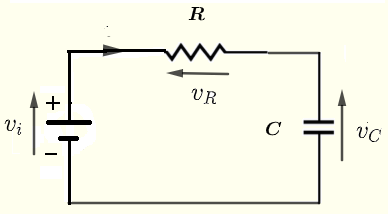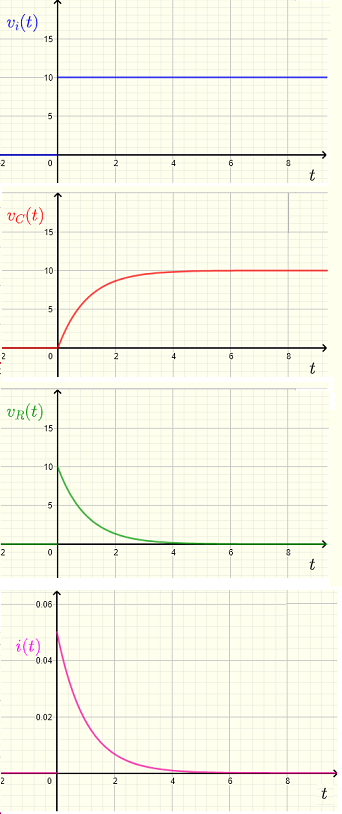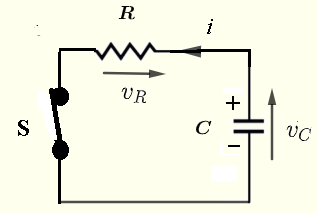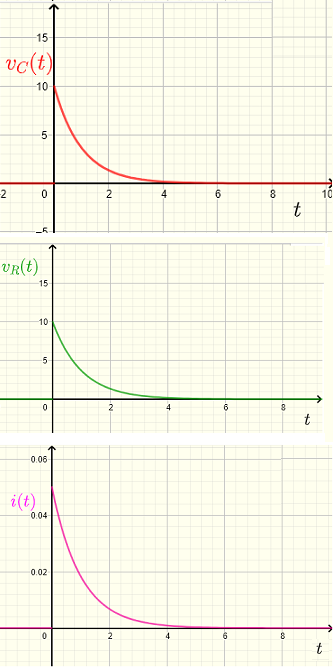# RC Circuit Response to a Step Voltage

Use of Laplace transforms to study the response of RC circuits to quick changes of the input voltage and currents is presented in the form of examples with detailed solutions. We also show how to mathematically model the charging and discharging processes of a capacitor. An online calculator to calculate expressions for voltages and current is also included.



## Problems with Solutions

Problem 1 Charging a capacitor
Find and graph the voltages across the capacitor $C$ and the resitor $R$ and the current $i$ as functions of time in the ciruit below given that the input voltage is $v_i = V_0 \; u(t)$, where $V_0 = 10$ V is a constant and $u(t)$ is the unit step function, the resistances $R = 200 \; \Omega$ and $C = 5$ mF. At $t = 0$, the voltage across the capacitor is equal to zero .Solution to Problem 1
Use Kirchhoff's law of voltages to write
$v_i(t) - v_R(t) - v_C(t) = 0$       (I)
Use Ohm's law to write
$v_R(t) = R \; i(t)$
Relationship between voltage and charging curent of a capacitor is given by
$\displaystyle v_C (t) = \dfrac{1}{C} \int i dt$
Take derivative of both side of the above and rewrite as
$i (t) = C \dfrac{d v_C}{dt}$
$v_R (t) = R i (t) = R C \dfrac{v_C}{dt}$
Hence equation (I) may be written as
$v_i (t) - R C \dfrac{v_C}{dt} - v_C (t) = 0$
Take the Laplace transform of both sides of the above equation
$\mathscr{L} \left \{ v_i (t) - R C \dfrac{v_C}{dt} - v_C (t) \right \} = \mathscr{L}\{ 0 \}$
Use the property of linearity of Laplace transform and aslo the fact that $\mathscr{L}\{ 0 \} = 0$ to rewrite the above as
$\mathscr{L} \left\{ v_i (t) \right\} - R\;C \mathscr{L} \left \{ \dfrac{v_C}{dt} \right \} - \mathscr{L} \left\{ v_C (t) \right \} = 0$       (II)
Let $\mathscr{L}\{ v_i (t) \} = V_i(s)$ and $\mathscr{L}\{ v_C (t) \} = V_C(s)$
Use the property of the derivative with respect to the time $t$ to rewrite (see formulas and properties of laplace transform)
$\mathscr{L} \left\{ \dfrac{v_C}{dt} \right \} = s V_C(s) - v_C(0)$
Given that at $t = 0$, the voltage across the capacitor is equal to zero, we have $v_C(0) = 0$ , equation (II) may be written as
$V_i(s) - R C s V_C(s) - V_C(s) = 0$
NOTE that we have transformed our initial differential equation from the time $t$ domain to the $s$ domain.
The Laplace transform $V_i(s)$ of $v_i(t) = V_0 u(t)$ is given by (see formulas and properties of laplace transform)
$V_i(s) = \dfrac{V_0}{s}$
The equation in the $s$ domain becomes
$R C s V_C(s) + V_C(s) = \dfrac{V_0}{s}$
Factor out $V_C(s)$ on the left side
$V_C(s) (R\;C\;s + 1) = \dfrac{V_0}{s}$
Solve for $V_C(s)$ to obtain
$V_C(s) = \dfrac{V_0}{s(R\;C s + 1)}$
Decompose the above into partial fractions to rewrite the above as ( see Appendix A at the bottom of the page for detailed calculations).
$V_C(s) = \dfrac{V_0}{s(R\;C s + 1)} = \dfrac{V_0}{s} - \dfrac{R\;C V_0}{R\;C s + 1}$
Divide numerator and denominator in the second term on the right side of the above equation by $R\;C$ and factor $V_0$ out to rewrite $V_C(s)$ as
$V_C(s) = V_0 \left(\dfrac{1}{s} - \dfrac{1}{s + \dfrac{1}{R\;C}} \right)$
We now use the formulas and properties of laplace transform to find the inverse Laplace transform $v_C(t)$ (time domain) of $V_C(s)$
$v_C(t) = V_0 \left( \mathscr{L^{-1}} \left\{ \dfrac{1}{s} \right\} - \mathscr{L^{-1}} \left\{ \dfrac{1}{s + \dfrac{1}{R\;C}} \right\} \right)$
$\mathscr{L^{-1}} \left\{ \dfrac{1}{s} \right\} = u(t)$
and
$\mathscr{L^{-1}} \left\{ \dfrac{1}{s + \dfrac{1}{R\;C}} \right\} = u(t) e^{-\frac{t}{R\;C}}$
Hence
$v_C(t) = V_0 (1 - e^{-\frac{t}{R\;C}} ) u(t)$
The voltage $v_R(t)$ across the resistor is given by
$v_R (t) = v_i - v_C = V_0 u(t) - V_0 u(t) (1 - e^{-\frac{t}{R\;C}} ) = V_0 e^{-\frac{t}{R\;C}} u(t)$
The current $i(t)$ is given by
$i(t) = \dfrac{v_R}{R} = \dfrac{V_0}{R} e^{-\frac{t}{R\;C}} u(t)$
Notes: The voltage $v_C(t)$ across the capacitor rises with time according to a natural exponential function $e^{-\frac{t}{R\;C}} )$ and hence the parameter $R\;C$ is called the time constant.

Numerical Applications
Let $V_0 = 10$ V , $R = 200 \; \Omega$ and $C = 5$ mF.
$R\;C = 200 \times 5 \times 10^{-3} = 1$ s (seconds)
$v_C(t) = 10 (1 - e^{-t} ) u(t)$ V
$v_R (t) = 10 e^{-t} u(t)$ V
$i(t) = 0.05 e^{-t} u(t)$ A
The graphs of the current and voltages are shown below.
Note the following at $t = 0$:
1) Because the capacitor was not charged prior to $t = 0$, the voltage $v_C(0)$ across the capacitor is equal to zero and the capacitor behaves like a short circuit at $t = 0$. $v_C(t)$ starts to increase as $t$ increases and this explains the charging process of the capacitor.
2) the voltage $v_R (0)$ across the resisitor is equal to the source voltage $10$ V and starts decreasing as $t$ increases.
3) The current $i(0)$ is at its maximum value $\dfrac{v_i(0) - v_C(0)}{R} = \dfrac{10 - 0}{200} = 10 / 200 = 0.05$ A and decreases as $t$ increases.
Note the following as $t$ is large:
$v_C(t)$ is almost equal to $v_i(t)$ meaning that the capacitor is fully charged. The current $i(t)$ is almost zero because the capacitor behaves like an open circuit .Problem 2 Discharging a capacitor
Capacitor $C$ in the circuit below is initially charged at $V_0 = 10$ Volts. At $t = 0$ the switch S in the circuit is closed. Find and graph the voltages across the capacitor $C$ and the resitor $R$, and the current $i$ as functions of time $t$.Solution to Problem 2
Use Kirchhoff's law of voltages to write
$v_C(t) - v_R (t) = 0$       (I)
Use Ohm's law to write
$v_R (t) = R \; i (t)$
The relationship between the voltage across a capacitor and the current through it is as follows:
Let $Q_0$ be the initial charge of the capacitor at $t=0$. Because the capacitor is discharging, the total charge will be decreasing and written as
$\displaystyle Q(t) = Q_0 - \int_0^{t} i(\tau) d\tau$
Voltage $v_C(t)$ across the capacitor is given by
$v_C(t) = \dfrac{Q(t)}{C} = \dfrac{Q_0}{C} - \dfrac{ \displaystyle \int i dt \ }{C }$
Take the derivative of the left side and the right side
$\dfrac{d v_C}{d t } = \dfrac{1}{C} \dfrac{d (Q_0 - \displaystyle \int i dt) }{d t }$
Use linearity of the derivative to write
$\dfrac{d v_C}{d t } = \dfrac{1}{C} \dfrac{d Q_0}{dt} - \dfrac{1}{C} \dfrac{d(\displaystyle \int i dt) }{d t }$
$Q_0$ is a constant and its derivative is equal to zero, hence the above may simplifies to
$\dfrac{d v_C}{d t } = - \dfrac{1}{C} i$
which may be written as
$i(t) = - C \; \dfrac{v_C}{dt}$     NOTE the minus sign is due to the fact that the capacitor is discharging .
Substitute $i(t)$ in $v_R (t)$ above to obtain
$v_R (t) = R \; i (t) = - R \; C \; \dfrac{d v_C}{dt}$
Hence equation (I) may be written as
$v_C (t) + R \; C \; \dfrac{d v_C}{dt} = 0$
Take the Laplace transform of both sides of the above equation
$\mathscr{L} \left \{ v_C (t) + R \; C \; \dfrac{d v_C}{dt} \right \} = \mathscr{L}\{ 0 \}$
Use the property of linearity of Laplace transform and aslo the fact that $\mathscr{L}\{ 0 \} = 0$ to rewrite the above as
$\mathscr{L} \left\{ v_C(t) \right \} + R\;C\;\mathscr{L} \left\{ \dfrac{d v_C}{dt} \right \} = 0$       (II)
Let $\mathscr{L}\{ v_C(t) \} = V_C(s)$
Use the property of the derivative with respect to the time $t$ to write (see formulas and properties of laplace transform)
$\mathscr{L} \left \{ \dfrac{d v_C}{dt} \right \} = s \; V_C(s) - v_C(0)$
Given that at $t = 0$, the capacitor is charged and its voltage is equal to $V_0$, we therefore have $v_C(0) = V_0$ ; and substituting in equation (II) to get
$V_C(s) + R \; C \; ( s \; V_C(s) - V_0 ) = 0$
Rewrite the above equation as
$V_C(s) \; (R\;C\;s + 1) = R \; C \; V_0$
Solve for $V_C(s)$ to obtain
$V_C(s) = \dfrac{R \; C \; V_0}{R \; C \; s + 1}$
Divide numerator and denominator by $R \; C$
$V_C(s) = \dfrac{ V_0}{s + \dfrac{1}{R\;C}}$
$v_C(t)$ is given by the inverse Laplace transform; hence
$v_C(t) = V_0 \left( \mathscr{L^{-1}} \left\{ \dfrac{1}{s + \dfrac{1}{R\;C}} \right\} \right)$
We now use the formulas and properties of laplace transform to find the inverse Laplace transform $v_C(t)$ (time domain) of $V_C(s)$
Hence
$v_C(t) = V_0 \; e^{-\frac{t}{R\;C}}$
The voltage $v_R(t)$ across the resistor is given by
$v_R (t) = v_C = V_0 \; e^{-\frac{t}{R\;C}}$
The current $i(t)$ is given by
$i(t) = \dfrac{v_R}{R} = \dfrac{V_0}{R} \; e^{-\frac{t}{R\;C}}$
Numerical applications: $V_0 = 10$ V , $R = 200 \; \Omega$ and $C = 5$ mF.
$R\;C = 200 \times 5 \times 10^{-3} = 1$ s (seconds)
$v_C(t) = 10 \; e^{-t}$ V
$v_R (t) = 10 \; e^{-t}$ V
$i(t) = 0.05 \; e^{-t}$ A
The graphs of the current and voltages are shown below.
Note the following at $t = 0$:
1) Because the capacitor was charged prior to $t = 0$ at the voltage $v_C(0) = 10$ Volts, it starts to decrease as $t$ increases and this explains the discharging process of the capacitor.
2) At $t = 0$; $v_R (0) = v_C(0) = 10$.
3) At $t = 0$, the current $i(0)$ is at its maximum given by $i(0) = \dfrac{v_R(0)}{R} = \dfrac{10}{200} = 10 / 200 = 0.05$ A and starts to decrease as $t$ increases.
Note the following as $t$ is large:
At large values of $t$, all voltages and the current are almost equal to zero because the capacitor has completely discharged and the energy that was stored in the capacitor is dissipated as heat by the resistor $R$.## Appendix A

Expand into partial fractions; find $A$ and $B$ such that
$\dfrac{V_0}{s(R\;C s + 1)} = \dfrac{A}{s} + \dfrac{B}{R\;C s + 1}$
Multiply all terms of the above by $s(R\;C s + 1)$ and simplify
$V_0 = A(R\;C\;s + 1) + B s$      (1)
Set $s = 0$ in equation (1) to obtain
$A = V_0$
Set $A = V_0$ and $s = 1$ in equation (1)
$V_0 = V_0 \times (R\;C \times 1 + 1) + B \times 1$
Simplify and solve for $B$
$B = - R\;C V_0$
Hence the decomposition into partial fractions
$\dfrac{V_0}{s(R\;C s + 1)} = \dfrac{V_0}{s} - \dfrac{R\;C V_0}{R\;C s + 1}$

## More References and Links

Solve Differential Equations Using Laplace Transform
Engineering Mathematics with Examples and Solutions